## Power Series

A power series in a variableis an infinite Sum of the form(1)

where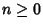and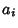are Integers, Real Numbers, Complex Numbers, or any other quantities of a given type.

A Conjecture of Pólya is that if a Function has a Power series with Integer Coefficients and Radius of Convergence 1, then either the Function is Rational or the Unit Circle is a natural boundary.

A generalized Power sumfor, 1, ... is given by(2)

with distinct Nonzero Roots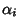, Coefficientswhich are Polynomials of degree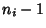for Positive Integers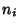, and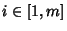. The generalized Power sum has order(3)

For any power series, one of the following is true:

1. The series converges only for.

2. The series converges absolutely for all.

3. The series converges absolutely for allin some finite open interval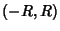and diverges ifor. At the pointsand, the series may converge absolutely, converge conditionally, or diverge.

To determine the interval of convergence, apply the Ratio Test for Absolute Convergence and solve for. A Power series may be differentiated or integrated within the interval of convergence. Convergent power series may be multiplied and divided (if there is no division by zero).(4)

Converges ifand Diverges if.

See also Binomial Series, Convergence Tests, Laurent Series, Maclaurin Series, Multinomial Series, p-Series, Polynomial, Power Set, Quotient-Difference Algorithm, Recurrence Sequence, Series, Series Reversion, Taylor Series

References

Arfken, G. Power Series.'' §5.7 in Mathematical Methods for Physicists, 3rd ed. Orlando, FL: Academic Press, pp. 313-321, 1985.

Myerson, G. and van der Poorten, A. J. Some Problems Concerning Recurrence Sequences.'' Amer. Math. Monthly 102, 698-705, 1995.

Pólya, G. Mathematics and Plausible Reasoning, Vol. 2: Patterns of Plausible Inference. Princeton, NJ: Princeton University Press, p. 46, 1954.